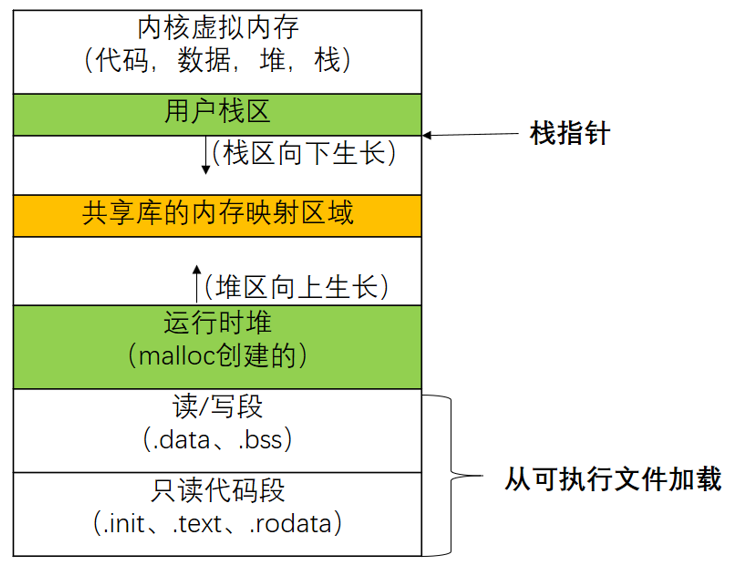# 从地址空间看进程和线程

## 一、什么是进程.bss是未初始化的全局和静态变量（不占空间，只是一个占位符）
.text是已经编译的程序机器代码

int a = 0; //全局初始化区
char *p1; //全局未初始化区
main()
{
int b; //栈
char s[] = "abc";// 栈
char *p2;// 栈
char *p3 = "123456"; //123456\0在常量区，p3在栈上。
static int c =0；// 全局（静态）初始化区
p1 = (char *)malloc(10);
p2 = (char *)malloc(20);
//分配得来得10和20字节的区域就在堆区。
strcpy(p1, "123456");// 123456\0放在常量区，编译器可能会将它与p3所指向的"123456"优化成一个地方。
}


## 二、什么是线程

#include <stdio.h>
#include <stdlib.h>
#include <pthread.h>
#define N 2

//线程函数
void *thread(void * vargp);
char **ptr;

int main()
{
int i;
pthread_t tid;
char *msg[N] = {"hello from foo",
"hello from bar"}; //主线程栈上定义的msg
ptr = msg;
for( i=0; i < N; i++)
pthread_create(&tid, NULL, thread, (void *)i);//创建两个对等线程
pthread_exit(NULL);
}

//线程函数
void *thread(void *vargp)
{
int myid = (int)vargp;
static int cnt = 0;
printf("[%d] : %s (cnt=%d)\n", myid, ptr[myid], ++cnt);//对等线程通过ptr引用主线程的msg，成功
return NULL;
}